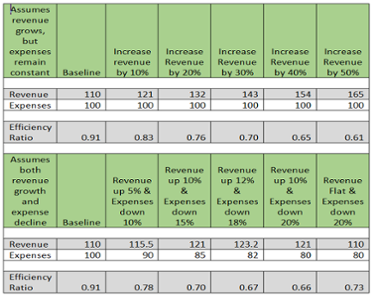•Financial Institutions (FIs) seek to operate at peak efficiency levels. For FIs, a fundamental ratio exists that helps define what operational efficiency should be, the Efficiency Ratio, which measures cost to revenue. It tells FIs how much money it spends to make a dollar of revenue. If a bank or credit union has an Efficiency Ratio of 0.75, it means the FI spends 75 cents to create every dollar of revenue.

An Efficiency Ratio is one way FIs compare themselves against their peers. Here are four ways that an Efficiency Ratio can be calculated:

1. Non-interest expense divided by total revenue less interest expense

2. Non-interest expense divided by net interest income before provision for loan losses

3. Non-interest expense divided by revenue

4. Operating expenses divided by fee income plus tax-equivalent net interest income

No matter how an organization calculates this rate, the premise is the same for everyone – the lower the number, the more efficient the organization. From an industry perspective, any organization between the 0.64 to 0.67 range is considered to be an efficient organization. If an FI’s Efficiency Ratio is above 0.67, they should be trying to find ways to improve efficiency.

The question then becomes “How do we go about improving this number?” Is it reducing expenses, increasing revenue, or perhaps doing some of both? The answer really depends on a number of factors that are impacting the institution, but in general, attacking both revenue and cost is important.

Example: Leveraging the Efficiency Ratio

FI A and FI B are located in the same geographical area, and compete with each other for business. In this example, one of FI A’s board members raised an issue about the Efficiency Ratio with management. Both FIs use equation three to calculate their Efficiency Ratio. FI A has revenue of 110 and expenses of 100. This yields an Efficiency Ratio of 0.91. The board member is concerned because FI B has an efficiency ratio of 0.66. FI A’s management looks at the numbers and realizes FI B’s revenue is 10 percent higher than theirs, or at 121. Since the FIs are in similar size, FI A assumes that costs must be similar in as well, so if they can improve their revenue by 10 percent and hold costs steady, they would be able to improve their Efficiency Ratio to be more competitive with FI B.

FI A’s strategy in the coming year is to increase revenue to match FI B. At the end of the year, FI A and FI B both have revenue of 121. However, when Efficiency Ratios are compared, FI A did improve from 0.91 to 0.83, but FI B has maintained its ratio at 0.66. FI A now realizes that despite the same market conditions, FI B has lower expenses than FI A. FI B has expenses of 80, or about 20 percent less than FI A.

At their current cost structure, FI A needs to increase revenue by about 40 percent to match FI B’s Efficiency Ratio. However, if FI A attacked both sides of the equation, increasing fees while decreasing expenses, the impact would have been far greater.

The table below illustrates how movement across both revenue and expense have a much greater impact than just moving the bar on one side. Generally, lowering the expense portion alone without impacting revenue will provide a greater improvement in the Efficiency Ratio than a similar increase in revenue. Illustrated below, increasing revenue by 20 percent and keeping costs flat results in a ratio of 0.76, versus reducing expenses by 20 percent but keeping revenue flat, yields a ratio of 0.73.

Table 1 – Efficiency Calculations with Different ScenariosUnderstanding operational efficiency is vital to a financial institution’s success, and by using the Efficiency Ratio as a measuring tool, banks and credit unions can strategically balance cost and revenue strategies to better optimize their operational efficiency.### John Mateker

Vice President
Hometown: Houston, Texas
Alma Mater: St. Mary’s University
Sports Fan, especially the San Antonio Spurs. Enjoys traveling and visiting historical sites, Reading, Early morning elliptical sessions.